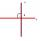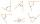# Slope

What is the slope of the line defined by the equation -2x +3y = -1 ?

Result

k =  0.67

#### Solution:Leave us a comment of example and its solution (i.e. if it is still somewhat unclear...):

Showing 0 comments:Be the first to comment!#### To solve this verbal math problem are needed these knowledge from mathematics:

Do you have a linear equation or system of equations and looking for its solution? Or do you have quadratic equation?

## Next similar examples:

1. V - slopeThe slope of the line whose equation is -3x -9 = 0 is
2. PerpendicularDetermine the slope of the line perpendicular to the line p: y = -x +4.
3. Supplementary anglesOne of the supplementary angles are three times larger than the other. What size is larger of supplementary angles?
4. Flowers 2Cha cruz has a garden. The ratio roses to tulips is 2 : 5, the ratio of roses to orchids is 7 : 6. Cha cruz wonders what the ratio of tulips to orchids is. If Cha cruz has 183 plants, how many of each kind are there?
5. Fifth of the numberThe fifth of the number is by 24 less than that number. What is the number?
6. Meanif the mean of the set of data 5, 17, 19, 14, 15, 17, 7, 11, 16, 19, 5, 5, 10, 8, 13, 14, 4, 2, 17, 11, x is -91.74, what is the value of x?
7. FractionFor what x expression ? equals zero?
8. Theorem proveWe want to prove the sentence: If the natural number n is divisible by six, then n is divisible by three. From what assumption we started?
9. LineIt is true that the lines that do not intersect are parallel?
10. BookAlena read a book at speed 15 pages per day. If she read twice as fast she should read a book four days earlier. How many pages have a book?
11. PineHow much of a mixed forest rangers want to cut down, if their head said: "We only cut down pine trees, which in our mixed forest 98%. After cut pine trees constitute 94% of all trees left."
12. Reciprocal equationDetermine the root of the equation: 9/x-7/x=1
13. RavensOn two trees sitting 17 ravens. If 3 ravens flew from first to second tree and 5 ravens took off from second tree then the first tree has 2 times more ravens than second tree. How many ravens was originally on every tree?
14. PearsAndrew, Lenka and Rasto have together 232 pears. Lenka has 28 more than Rasto and Rasto pears have 96 more than Andrew. Determine how much each of them has pears.
15. Boxes200 boxes have been straightened in three rows. The first was 13 more than in the second, and in the second was one fifth more than in the third one. How many boxes are in each row?
16. Equation with absSolve this equation with absolute value member: ?
17. GlassTrader ordered from the manufacturer 200 cut glass. The manufacturer confirmed the order that the glass in boxes sent a kit containing either four or six glasses. Total sent 41 boxes. a) How many boxes will contain only 4 glasses? b) How many boxes will co